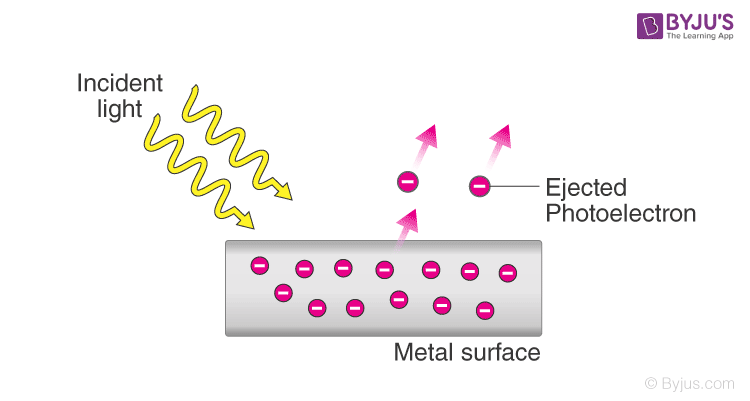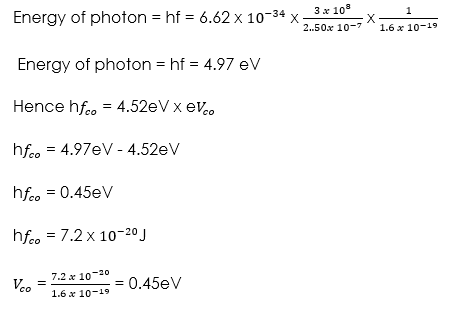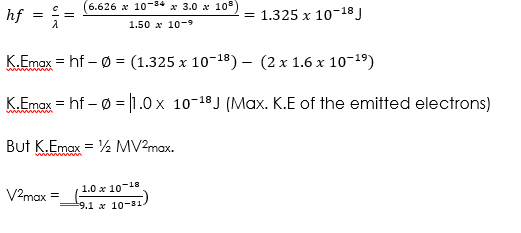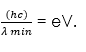# Photoelectric Effect

Welcome to class!

In today’s class, we will be talking about the photoelectric effect. Enjoy the class!

### Photoelectric EffectThe photoelectric effect was discovered by Heinrich Hertz in 1887. The photoelectric effect is a phenomenon in which electrons are emitted from the surface of a substance when certain electromagnetic radiation falls on it.

Metal surfaces require ultra-violet radiation while caesium oxide needs a visible light i.e. optical spectrum (sunlight).

#### Work function

A minimum amount of work is needed to remove an electron from its energy level to overcome the forces binding it to the surface.

This work is known as the work function with units of electron volts (eV). One electron volt is the work done when one electron is transferred between points with a potential difference of one volt; that is,

1 eV = 1 electron × 1 volt

1 eV = 1.6 × 10-19 × 1 volt

1 eV = 1.6× 10-19 Joules (J)

Hence: E = eV = hf – hf0

#### Threshold frequency

This is the minimum frequency of the radiation that will cause a photoelectric effect on a certain surface. The higher the work function, the higher the threshold frequency.

##### Factors affecting the photoelectric effect
1. The intensity of the incident radiation: the rate of emission of photoelectrons is directly proportional to the intensity of incident radiation.
2. The work function of the surface: photoelectrons are emitted at different velocities with the maximum being processed by the ones at the surface.
##### Planck’s constant

Max Planck’s predicted the energy of an oscillating atom to be E = nhf, where n – integer, f – frequency of the source, h – Planck’s constant which has a value of 6.63 × 10-34 Js.

Einstein proposed that light is made up of packets of energy called photons which have no mass but they have momentum and energy given by;The number of photons per unit area of the cross-section of a beam of light is proportional to its intensity. However, the energy of a photon is proportional to its frequency and not the intensity of the light.

##### Einstein’s photoelectric equation

As an electron escapes energy equivalent to the work function ‘Φ’ of the emitter substance is given up. So the photon energy ‘h f’ must be greater than or equal to Φ. If the ‘hf’ is greater than Φ then the electron acquires some kinetic energy after leaving the surface.

The maximum kinetic energy of the ejected photoelectron is given by;

K.Emax = ½ mv2max = hf – Φ ……………… (i),

Where mv2max = maximum velocity and mass.

##### This is Einstein’s photoelectric equation.

If the photon energy is just equivalent to work function then, m v2max = 0, at this juncture the electron will not be able to move hence any photoelectric current, giving rise to a condition known as cut-off frequency, hfco = Φ………………. (ii)

Also, the p.d required to stop the fastest photoelectron is the cut-off potential, V co which is given by E = e V co electron volts, but this energy is the maximum kinetic energy of the photoelectrons and therefore,

½ m v2max = e V co ………….. (iii).

Combining equations (i), (ii) and (iii), we can write Einstein’s photoelectric equation as, eV co = hf – hfco ………………….. (iv)

NOTE: — Equations (i) and (iv) are quite useful in solving problems involving photoelectric effect.

###### Worked examples

(1) The work function of tungsten is 4.52eV. Find the cut-off potential for photoelectrons when a tungsten surface is illuminated with radiation of wavelength 2.50 x m. (Planck’s constant, h = 6.62 x Js).

Solution(2) Sodium has a work function of 2.0eV. Calculate

(a) The maximum energy and velocity of the emitted electrons when sodium is illuminated by a radiation of wavelength 150 nm.

(b) Determine the least frequency of radiation by which electrons are emitted. (Take ‘h’ = 6.626 x Js, e = 1.6 x , c = 3.0 x m/s and mass of electron = 9.1 x kg).

Solution##### X-rays

X-rays were discovered by a German scientist named Roentgen in 1895. They can pass through most substances including soft tissues of the body but not through bones and most metals. They were named X-rays meaning ‘unknown rays’.

###### X-ray production

They are produced by modified discharge tubes called X-ray tubes. The cathode is in the form of a filament which emits electrons on heating.

The anode is made of solid copper molybdenum and is called the target. A high potential difference between the anode and the cathode is maintained by an external source. The filament is made up of tungsten and coiled to provide high resistance to the current. The lead shield absorbs stray x-rays.

###### Energy changes in an X-ray tube.

When the cathode is heated electrons are emitted by thermionic emission. They acquire electrical energy which can be expressed as E = e V. Once in motion the electrical energy is converted to kinetic energy, that is eV = ½ mev2.

The energy of an electromagnetic wave can be calculated using the following equation Energy = hf, where h- Planck’s constant, f – frequency of the wave.

The highest frequency of the X-rays released after an electron hits the target is when the greatest kinetic energy is lost, that is hf max = eV.

Lower frequencies are released when the electrons make multiple collisions losing energy in stages, the minimum wavelength, λ min, of the emitted X-rays is given by;These expressions can be used to calculate the energy, frequencies and wavelengths of X-rays.

###### Properties of X-rays
1. They travel in straight lines
2. They undergo reflection and diffraction
3. They are not affected by electric or magnetic fields since they are not charged particles.
4. They ionize gases causing them to conduct electricity
5. They affect photographic films
###### Hard X-rays

These are x-rays on the lower end of their range (10-11 – 10-8 m) and have more penetrating power than normal x-rays.

They are capable of penetrating flesh but are absorbed by bones.

###### Soft X-rays

They are on the upper end of the range and are less penetrative. They can only penetrate soft flesh and can be used to show malignant growth in tissues.

###### Dangers of X-rays and the precautions
1. They can destroy or damage living cells when overexposed.
2. Excessive exposure of living cells can lead to a genetic mutation.
3. As a precautionary measure X-ray tubes are shielded by lead shields.
###### Uses of X-rays
1. Medicine: X-ray photos called radiographs are used as diagnostic tools for various diseases. They are also used to treat cancer in radiotherapy.
2. Industry: they are used to photograph and reveal hidden flaws i.e. cracks in metal casting and welded joints.
3. Security: used in military and airport installations to detect dangerous metallic objects i.e. guns, explosives, grenades etc.
###### General evaluation
1. State and explain Einstein’s Photoelectric equation.

Assignment

1. Calculate the frequency of the photon whose energy required to eject a surface electron with a kinetic function of 1.97 x 1016 eV if the work function of the metal is 1.33 x 10-16eV

[1 eV = 1.6 x 10-19J, h = 6.6 x 10-34Js]

In our next class, we will be talking about Conduction of Electricity Through Gases. We hope you enjoyed the class.

Should you have any further question, feel free to ask in the comment section below and trust us to respond as soon as possible.

How Can We Make ClassNotesNG Better - CLICK to Tell Us💃